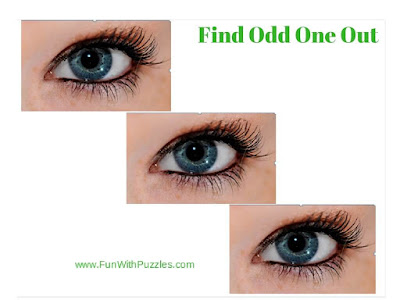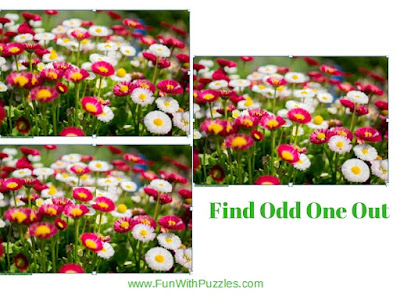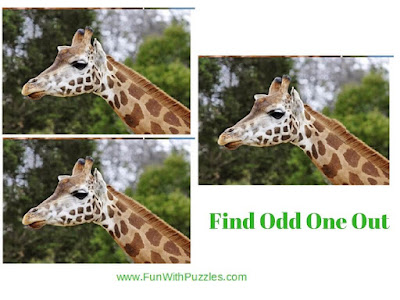Recently we have published Odd one Out Number Puzzles which were very much loved by our audience. In those puzzles, one has to find a number that is different from others among the given set of numbers. However Visual Picture Puzzles are different and are very interesting puzzle types. Kids will love to solve these puzzles as these puzzles do not require any knowledge of mathematical calculations. Also, these puzzles are language-neutral puzzles. It means to solve these puzzles, not specific language knowledge is required. We have already published Odd One Out Picture Puzzles for Kids which were an easy one. Today's Picture Puzzles are tough and hence recommended only for teens and adults as kids may find these puzzles very difficult.
Finding the Odd One Out among the given pictures is a very interesting visual picture puzzle. In these puzzles, three pictures are given. On the first look, all these three pictures will look exactly alike. However, two of these pictures are exactly the same and the third one is different slightly from the other two pictures. One has to spot this difference between the one picture from the others in these pictures.
Here in this post, I am putting 5 different puzzles. Answer links to these puzzles are given next to each puzzle. However, try not to look at the answers immediately. Don't give up easily and try to solve these picture puzzles as quickly as possible.1. Can you spot which one is different?2. Can you find which one is different?3. Can you find which is the Odd One Out Picture?4. Can you find which two pictures are exactly similar in this puzzle?

The next Spot the Difference puzzle is the toughest of all these puzzles. Try not to look at the answer to this puzzle. Give your 100% before looking at the answer to this puzzle.5. Can you solve this tough Odd One Out Picture Puzzle?

Do try out similar Odd One Out Puzzles as mentioned below
List of Odd One Out Puzzles and Brain Teasers

1. Picture Puzzles for Genius Minds: These are tough Odd One Out Puzzles only for Genius Mind in which one has to find which picture is different out of four given pictures.

2. Odd One Out - Fun Letters Quiz for Kids: These are the Odd One Out Puzzles in which some letters are given in each of the Picture Puzzles and your challenge is to find which letter is Odd One Out.

3. Spot the Different Picture Brain Teasers for teens with answers: In these Picture Puzzles, three images are given. In these images, two images are exactly alike and only one is different. Your challenge is to spot which picture is different from the other three puzzle images.

4. Picture Puzzles for Teens With Answers: Here you can only find Odd One Out Picture Puzzles, but also other types of picture puzzles e.g. Puzzle in which one has to find the hidden letter or hidden animals.

#### 1 comment:

L&V said...

Answer is 20. Add the top left number (11) and bottom left number (4) and you get 15. Add the two left middle numbers (7 and 8) and they total 15. Therefore, when you add the two middle right numbers (16 and 17), they total 33. So the top right and bottom right numbers must total 33. 13 plus 20 equals 33.